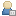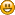###Show Posts

This section allows you to view all posts made by this member. Note that you can only see posts made in areas you currently have access to.

### Messages - Guerrilla705

Pages: 
1
##### Releases / Re: 2D Toolkit 2.3.0
« on: November 14, 2013, 02:42:34 am »
How should I go about getting the updated 2.3 from having 2.2? It's been a while since I got 2.2, so I don't remember where my invoice is to register on this forum. Is there any way to download 2.3 when I already have 2.2 set up and running? Thanks

2
##### Support / Re: Raycasts with tilemap mesh colliders
« on: August 20, 2013, 08:05:11 pm »
I suppose not with the raycasting problem. The rigid body is needed to make the box collider on the character non static, then the kinematic so it doesn't have physics effects applied. I suppose I just wanted a way around the capsule collider of the character controller, while still having easy collision detection available. The kinematic rb collider will not send collision messages with the static collider of the tilemap to my understanding, so I needed to use raycasting instead for that collision detection. I cannot seem to get raycasts to correctly return true when hitting the tilemap mesh colliders. perhaps I am simply missing something simple and unrelated

3
##### Support / Re: Raycasts with tilemap mesh colliders
« on: August 20, 2013, 06:30:31 pm »
Yea, the rays are definitely starting outside the tilemap (even put to an extreme for testing). Could it be that static colliders (like the ones on the tilemap chunks) don't collide with kinematic rigidbody colliders?

4
##### Support / Raycasts with tilemap mesh colliders
« on: August 20, 2013, 12:59:01 am »
(First post, sorry if it is too noobish :c)

Hey guys and gals! I am working on a 2d platformer project, and as many have seen before, the character controller's forced capsule collider just doesn't cut it. I have taken to using a kinematic rigid body along with a box collider (for a more retro style hitbox), and I am currently trying to deal with collisions using raycasts.

At the moment I have a ray that points down and extends very slightly lower than the box collider, and I am using Physics.Raycast in place of controller.isGrounded to check for collisions (and also to allow different responses to touching things with the RaycastHit inclusion). However, I cannot seem to get the Raycast to catch correctly when it hits the tilemap render data chunk colliders from my tk2d tilemap. I was wondering if anyone has seen this problem before, or has a better solution to the character controller capsule collider problem? (code below)

Thanks in advanceCode: [Select]
`using UnityEngine;using System.Collections;public class BleakController : MonoBehaviour { //public vars public Rigidbody rigidBody; public BoxCollider boxCollider; public GameObject tileMapRenderData; public int runSpeed = 350; public float joggingMultiplier = .6f; public int jumpSpeed = 250; public int maxFallSpeed = 400; public int gravityAcceleration = 600; public float slamMultiplayer = 1.25f; public bool canControl = true; public Transform startPoint; public float timeUntilRun = 1.75f; public const float SMALL_JUMP_TIME = .06f; public const float MED_JUMP_TIME = .1f; public const float LARGE_JUMP_TIME = .12f; //private vars private bool idleSwitch = true; private float idleDelay = 0f; private float runDelay = 0f; private float slamDelay = 0f; private float jumping = 0f; private bool slamming = false; private bool slamLanding = false; private Vector3 velocity = new Vector3 (0,0,0); //private Vector3 moveDelta = new Vector3 (0,0,0); private bool facing = true; private Ray downRay; private float downRayLength; private RaycastHit downRayHit; private const bool LEFT = false; private const bool RIGHT = true; private float dt; // Use this for initialization void Start () { transform.position = startPoint.position; rigidbody.isKinematic = true; downRay = new Ray(rigidBody.position, Vector3.down); downRayLength = (boxCollider.size.y/2)+0.1f; } // Update is called once per frame void Update () { downRay.origin = rigidBody.position; Debug.DrawRay(downRay.origin,Vector3.down*downRayLength,Color.green); velocity.x = 0; dt = Time.deltaTime; if (canControl){ if (!Physics.Raycast(downRay, out downRayHit, downRayLength)){ velocity.y -= gravityAcceleration * dt; } else { Debug.Log ("hitting something"); //deal with hitting objects from the top } UpdateIdle(dt); UpdateLeftRight(dt); UpdateJump(dt); } else { //accel y is 0, so velocity.y shouldn't change. walking up stairs and such } UpdateRotation(dt); UpdateRigidBodyPosition(dt); if (Debug.isDebugBuild) UpdateDebug(dt); } /// <summary> /// Updates the idle animations. /// </summary> /// <param name='dt'> /// time since last frame was called (in seconds) /// </param> void UpdateIdle(float dt){ if (jumping>=0){ //handle idle animations if (idleDelay >= 7) idleDelay = 0; else{ if (Input.GetAxisRaw("Horizontal") == 0 && !Input.GetKey(KeyCode.Space) && idleDelay <=6){ //play idle1 idleDelay += dt; runDelay = 0; } else if (Input.GetAxisRaw("Horizontal") == 0 && !Input.GetKey(KeyCode.Space)){ switch (idleSwitch){ case true: //play idle2 idleSwitch=false; break; case false: //play idle3 idleSwitch=true; break; } idleDelay += dt; runDelay = 0; } } } } /// <summary> /// Updates x movement based on controls. /// </summary> /// <param name='dt'> /// time since last frame was called (in seconds) /// </param> void UpdateLeftRight(float dt){ //left and right movement float directionInt = Input.GetAxisRaw("Horizontal"); if (directionInt < 0) facing = LEFT; if (directionInt >0) facing = RIGHT; //player is hitting direction if (directionInt != 0){ idleDelay = 0; if (runDelay < timeUntilRun){ velocity.x = runSpeed * joggingMultiplier * directionInt; if (jumping != -1){ //play jogging anim } runDelay += dt; } else { velocity.x = runSpeed * directionInt; if (jumping != -1){ //play running anim } } } else { //otherwise horizontal direction isn't being applied runDelay = 0; } } /// <summary> /// Updates jump logic (including slams) /// </summary> /// <param name='dt'> /// time since last frame was called (in seconds) /// </param> void UpdateJump(float dt){ if (Input.GetKeyDown(KeyCode.Space) && jumping >= 0){ //play jump sound jumping += dt; if (jumping >= LARGE_JUMP_TIME) jumping = -1; } else { jumping = -1; } if (jumping > 0){ if (jumping < SMALL_JUMP_TIME) velocity.y = jumpSpeed/2; else if (jumping < MED_JUMP_TIME) velocity.y = jumpSpeed*2/3; else velocity.y = jumpSpeed; } if (Physics.Raycast(downRay, out downRayHit, downRayLength)){ if (slamming == true) slamLanding = true; slamming = false; jumping = 0; } //beginning a slam if (jumping == -1 && Input.GetKeyDown(KeyCode.LeftShift)){ velocity.y = -jumpSpeed * slamMultiplayer; slamming = true; //play slamming animation and sound } //landing from a slam if (slamLanding){ slamLanding=false; slamDelay=0; runDelay=0; } } /// <summary> /// Updates rotation /// </summary> /// <param name='dt'> /// time since last frame was called (in seconds) /// </param> void UpdateRotation(float dt){ Debug.Log (transform.rotation.y); if (!facing && transform.rotation.y <= 0.1f){ transform.Rotate(0,180,0); } if (facing && transform.rotation.y >= .9f){ transform.Rotate (0,180,0); } } /// <summary> /// Updates the rigid body's velocity in accordance with the local velocity var /// </summary> /// <param name='dt'> /// time since last frame was called (in seconds) /// </param> void UpdateRigidBodyPosition(float dt){ velocity.z=0; rigidbody.MovePosition(rigidBody.position + velocity*dt); } /// <summary> /// Update step for any debugging happening /// </summary> /// <param name='dt'> /// time since last frame was called (in seconds) /// </param> void UpdateDebug(float dt){ if (Input.GetKeyDown (KeyCode.Q)) Debug.DebugBreak(); }}`

Pages: